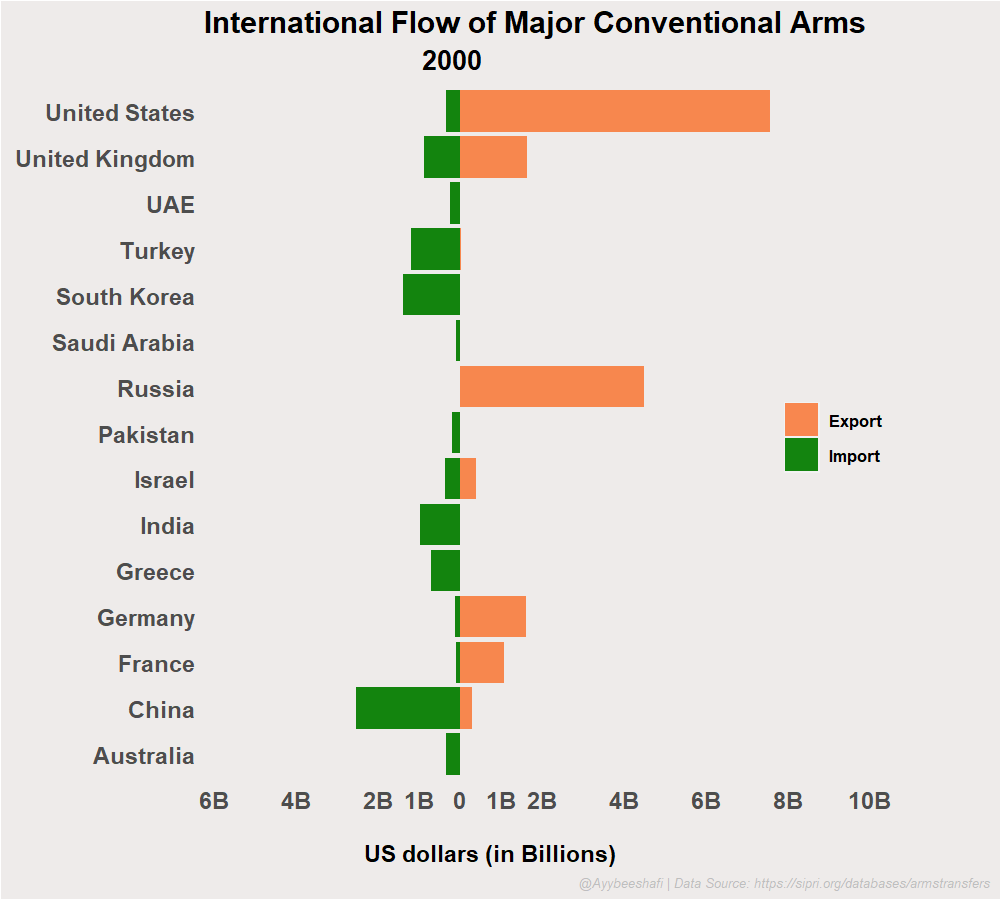# How to make animated Stacked Bar chart of Arms Export by Countries via R and GGPLOT

H

A guide to create a stacked bar chart or bar graph of significant arms and weaponry transfers between countries in the past year. This guide will use R, GGPLOT, GG_Animate, and gifski. In addition, we aim to use the conventional weapons transfer data from Stockholm International Peace Research Institute and create an animated stacked bar chart of arms export and import.

### First, to install the required libraries:

```
if(!require('pacman')) install.packages('pacman')

```

It will install all the required libraries for the required graph.

Now import the libraries in the program.

```library(tidyverse)
library(ggpol)
library(gganimate)
library(gifski)
```

The next thing is downloading the data from the SIPRI Arms Transfer Database. It has all the information on transfers of international transfer of ammunition and conventional weapons between countries. We need this data to get insight into how much US dollars are being spent on importing and exporting firearms around the world.

1. Now, let’s process the data to make it suitable for our visualization. After loading the CSV file, we remove all the empty fields in the CSV file.
2. Next, we separate the Imports from exports and factor them accordingly.
3. The next step is to remove the columns that are not necessary and use the Year, Sales, and Country columns.
4. In order to plot the Imports on the same bar chart but on the left side of zero, we multiply all the entries with imports by -1
```

IEPop &amp;lt;- IEpop %&amp;gt;%

na.omit %&amp;gt;%

mutate(ImportorExport = as.factor(ifelse(str_detect(IOE, 'Export\$') == TRUE, 'Export', 'Import')),

ImportorExport = factor(ImportorExport, levels = c('Export', 'Import'))) %&amp;gt;%

select(-Total,-IOE) %&amp;gt;%

gather(Year, Sales, X2000:X2020) %&amp;gt;%

mutate(Year = as.integer(substr(Year, 2, 5)),
Sales = ifelse(ImportorExport == 'Import', as.integer(Sales * -1), as.integer(Sales)))

```

## The next step is to plot the static stacked bar chart or Pyramid.```
PopPyramid &amp;lt;- IEPop %&amp;gt;%

ggplot(aes( x = Country, y = Sales, fill = ImportorExport, ) )

+ geom_bar(stat = 'identity')

+ scale_fill_manual(values = c('#f8874f', '#13840f'))

+ coord_flip()

+ scale_y_continuous( breaks = c(-1000,-2000,-4000,-6000,-8000,-10000, 0,1000,2000,4000,6000,8000,10000),

label = c("1B","2B","4B","6B","8B","10B","0","1B","2B","4B","6B","8B","10B") )

```

Now we will set the theme according to our liking and aesthetics.

```
PopPyramid <- PopPyramid +
theme(
legend.position = c(0.8,0.5),
plot.background = element_rect(fill = "#eeebea"),
axis.ticks = element_blank(),
axis.title.y = element_blank(),
legend.title = element_blank(),
panel.background = element_blank(),
panel.border = element_blank(),
strip.background = element_blank(),
strip.text.x = element_blank(),
panel.grid.minor = element_blank(),
panel.grid.major = element_blank(),
axis.text = element_text(size = 14,face = 'bold'),
legend.key.size = unit(0.75, 'cm'),
legend.background = element_rect(fill = "#eeebea"),
legend.text = element_text(
size = 10,
hjust = 0,
face = 'bold'
),
plot.title = element_text(
size =18,
hjust = 0,
face = 'bold'
),
plot.subtitle = element_text(
size = 16,
hjust = 0.3,
face = 'bold'
),
axis.title.x = element_text(
hjust = 0.3,
size = 14,
face = 'bold'
),
plot.caption = element_text(
size = 8,
hjust = 1,
face = 'italic',
color = 'gray'
)
)
```

In the following code, we will set the labels and captions for the animated stacked bar chart of arms export and import by country.

```
PopPyramid <- PopPyramid

+ labs( title = 'International Flow of Major Conventional Arms\n',

subtitle = '{closest_state}',

y = '\n\nUS dollars (in Billions)',

caption = '@Ayybeeshafi | Data Source: sipri org/databases/armstransfers' )

```

The last step is to set the Bar Graph of Arms Export animation and create a GIF of the transfer of conventional weapons from 2000 to 2020.

```
PopPyramid <- PopPyramid +
transition_states(
Year,
transition_length = 1,
state_length = 2,
wrap = FALSE
) +
ease_aes('cubic-in-out')
animate(
PopPyramid,
fps = 25,
duration = 20,
width = 1000,
height = 900,
res = 120,
end_pause = 80,
renderer = gifski_renderer('ArmsImportExport.gif')
)

```

Datasource can be found here.

I hope this guide was helpful to you. Suppose you have any questions or queries regarding how to create a population pyramid or stacked bar chart of arms import and export. Then, write to us in the comments.